Get instant live expert help with Excel or Google Sheets“My Excelchat expert helped me in less than 20 minutes, saving me what would have been 5 hours of work!”

#### Post your problem and you’ll get Expert help in seconds.

Your message must be at least 40 characters
Our professional Expert are available now. Your privacy is guaranteed.

# How to Get Excel to Stop Rounding Your Numbers

Isn’t it annoying when Excel just keeps rounding off numbers?  Below table shows some common examples: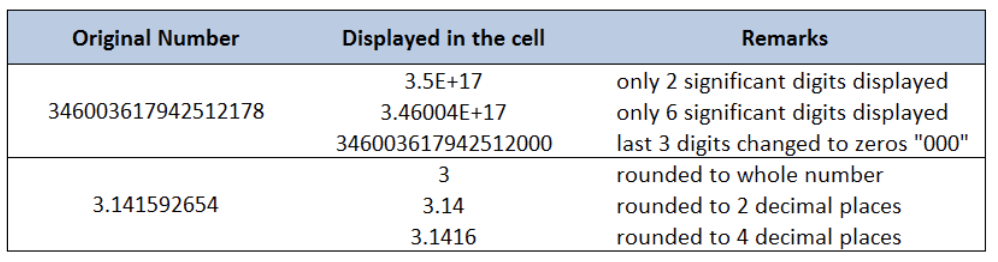Figure 1. Examples of Excel Rounding Off Numbers

There are several possible reasons but the most common are these:

• Column width too narrow and doesn’t display the whole number
• The number of decimal places is set to fewer digits than the actual decimal places
• The number is too large and exceeds 15 digits; Excel is limited to display only 15 significant digits
• The default format for every cell in Excel is set to “General” and not “Number”

• Stop Excel From Rounding Large Numbers
• Stop Excel From Rounding Whole Numbers
• Stop Excel From Rounding Currency

## Stop Excel From Rounding Large Numbers

There are instances when we need to enter large numbers, such as credit card or reference numbers. Unfortunately, Excel has a limitation and displays only 15 significant digits. The excess digits will be changed to zeros.

Example: In cell D3, enter the number “346003617942512178”, which contains 18 digits.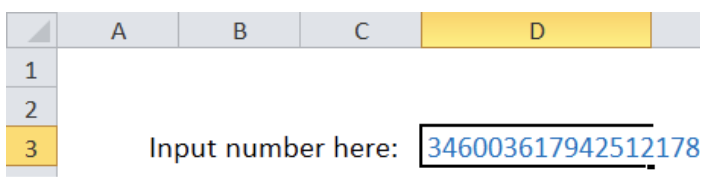Figure 2.  Entering an 18-digit number in Excel

Press enter.  Cell D2 will display the value “3.46004E+17” while the formula bar will display the value “346003617942512000”.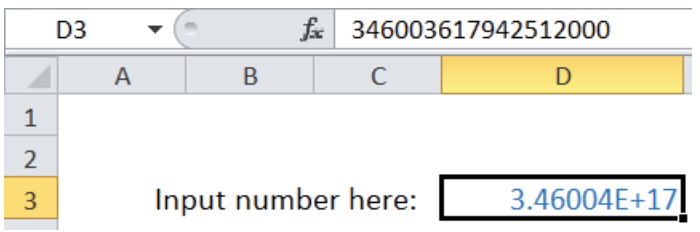Figure 3. Excel rounding a large number and showing 15 significant digits only

Notes:

• The 16th to 18th digits are changed from “178” to “000”.
• The displayed value only shows 6 significant digits because default format in Excel is “General”
• To display the 18-digit number 346003617942512000, select D3 and press Ctrl + 1 to launch the Format Cells dialog box.  Select the format “Number” and set the decimal places to zero “0”.  The 18-digit number is now displayed in D3.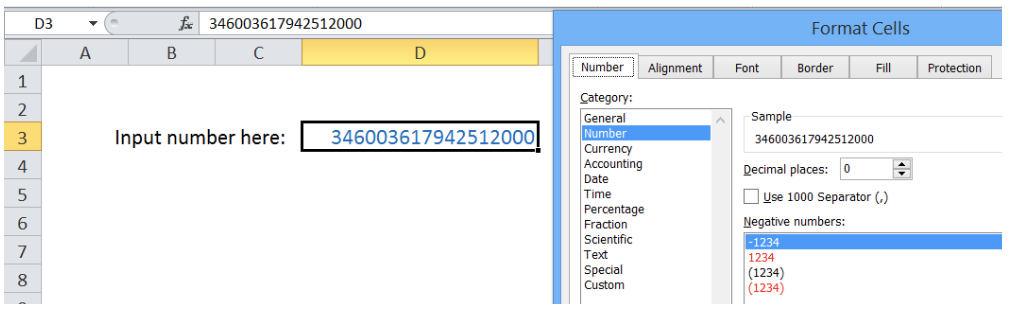Figure 4. Changing the cell format from “General” to “Number”

But how to display the original number 346003617942512178?

Work-around:

To stop Excel from rounding a large number, especially those exceeding 15 digits, we can:

• Format the cell as text before entering the number; or
• Enter the number as a text by entering an apostrophe “ before the number
• Example: Enter into cell D3: ‘346003617942512178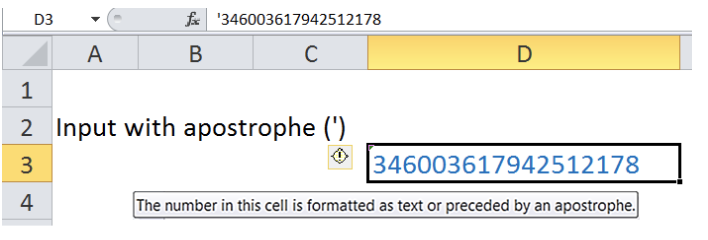Figure 5. Entering the large number as text by preceding with an apostrophe

## Stop Excel From Rounding Whole Numbers

Let’s take for example the value of pi(), which is commonly used in mathematical equations.

Example 1 : In cell D3, enter the formula =pi(). Column E displays the value of pi with varying number of decimal places.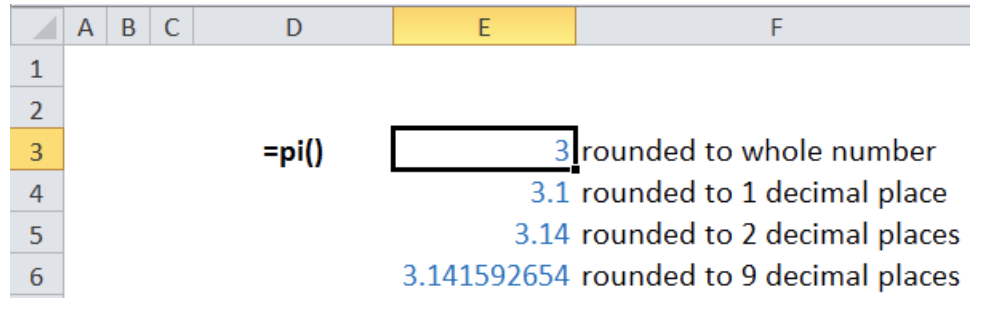Figure 6. The value of pi() displayed with varying number of decimal places

Work-around:

To stop Excel from rounding whole numbers, click the Increase Decimal button in the Home > Number tab.  Increase the decimal place until the desired number of decimal places is displayed.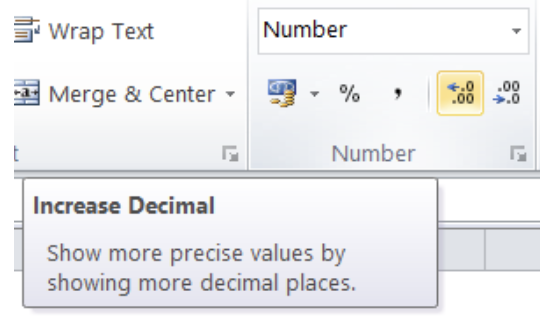Figure 7. Increase Decimal button in Excel

Example 2: In cell D3, enter the number 123456789, and see how Excel rounds off the number into varying number of significant digits, depending on the column width.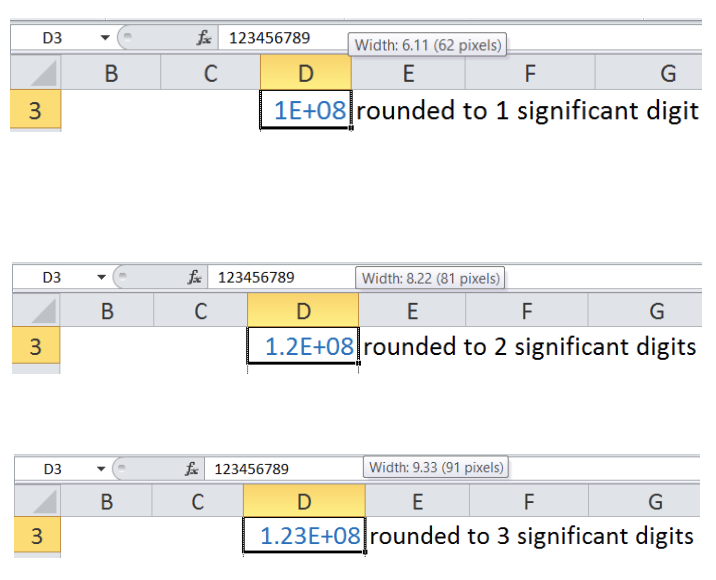Figure 8.  A large number displayed with different number of significant digits based on column width

Work-around:

To stop Excel from rounding a whole number, we can adjust the column width to display all the digits.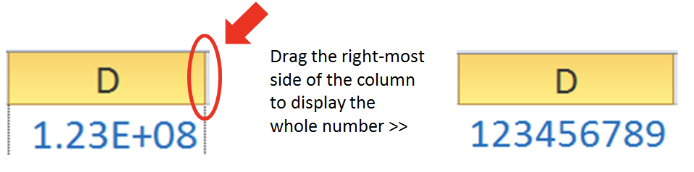Figure 9. Adjusting column width to display the entire number

## Stop Excel From Rounding Currency

Most currencies have two decimal places. Rounding currencies might have a small impact since it will only be dealing with tenths or hundredths of a currency, such as centavos.

There are instances, however, where rounding currencies becomes serious. In Accounting, for example, in order to arrive at a more accurate result, rounding should be done only as needed, or as late into the calculations as possible.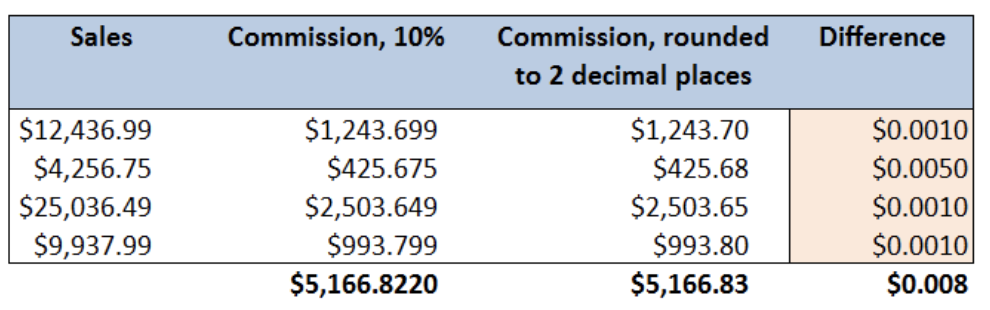Figure 10.  Sample calculation showing the difference with rounded numbers

The table above shows the effect of rounding currencies into the total commission.

Work-around:

To stop Excel from rounding currencies, format the decimal places to “3 or more”.  This way, the precision and accuracy of our data is preserved as close to the original value as possible.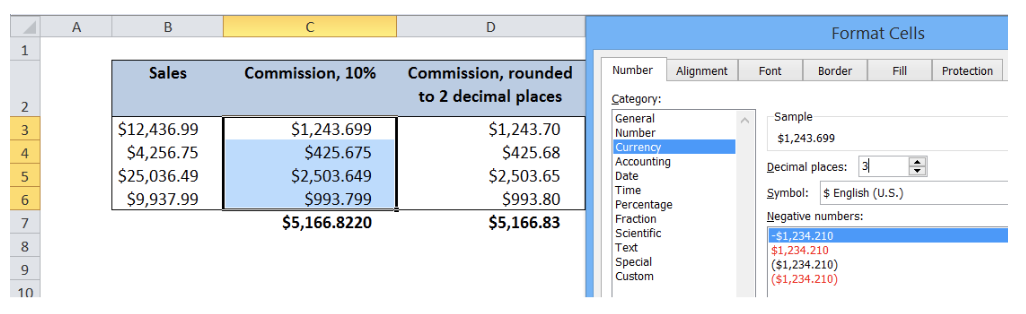Figure 11. Setting the decimal places to display the whole value of currencies

Most of the time, the problem you will need to solve will be more complex than a simple application of a formula or function. If you want to save hours of research and frustration, try our live Excelchat service! Our Excel Experts are available 24/7 to answer any Excel question you may have. We guarantee a connection within 30 seconds and a customized solution within 20 minutes.

### Did this post not answer your question? Get a solution from connecting with the expert.Another blog reader asked this question today on Excelchat:## Subscribe to Excelchat.coAnother blog reader asked this question today on Excelchat: